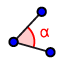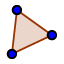# Triangle Congruence: SAS

## Construct a triangle with an angle of 70° between sides of lengths 4 and 7.

1. Select the SEGMENT WITH GIVEN LENGTHtool, and enter a length of 4. 2. With the SEGMENT WITH GIVEN LENGTHtool still selected, click on point A, and enter a length of 7. 3. Select the MOVEtool, and drag point C so that you can see both segments. 4. Select the ANGLEtool. Click points B, A, and C to measure angle A. If the measurement is over 180°, undo and click on them in reverse order. 4. Select the MOVEtool, and move point C until angle BAC has a measure of 70°. 5. Select the DISTANCE OR LENGTHtool, and then click on points C and B to measure the distance between them. 6. If you want to see your triangle more clearly, select the POLYGONtool, and then click on A, B, C, and A again. 7. Answer the questions below the applet by constructing each triangle describe below.

Construct a triangle with an angle of 70° between sides of lengths 4 and 7. What are the measures of the other angle, side, and angle?

Construct a triangle with an angle of 130° between sides of lengths 2 and 5. What are the measures of the other angle, side, and angle?